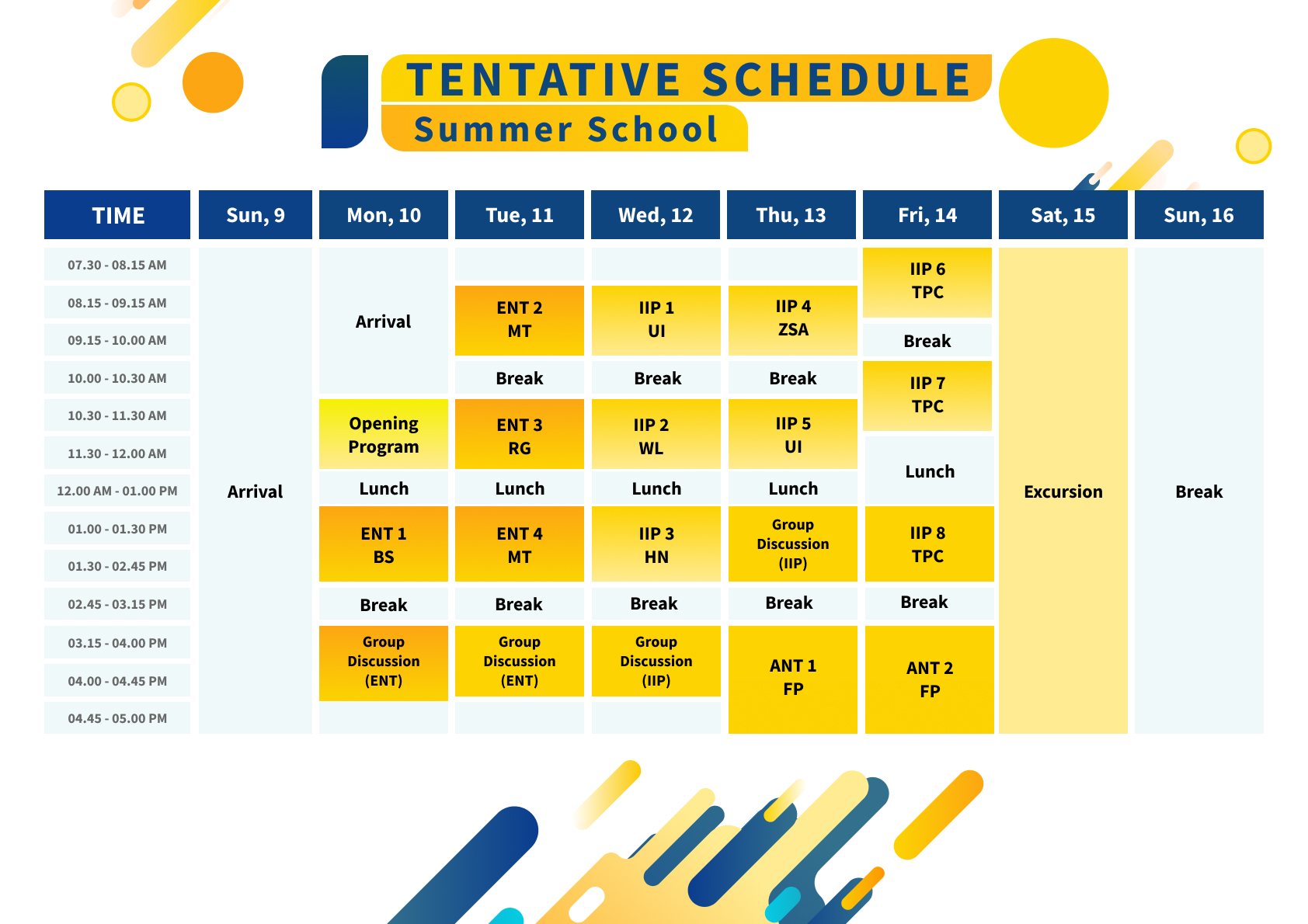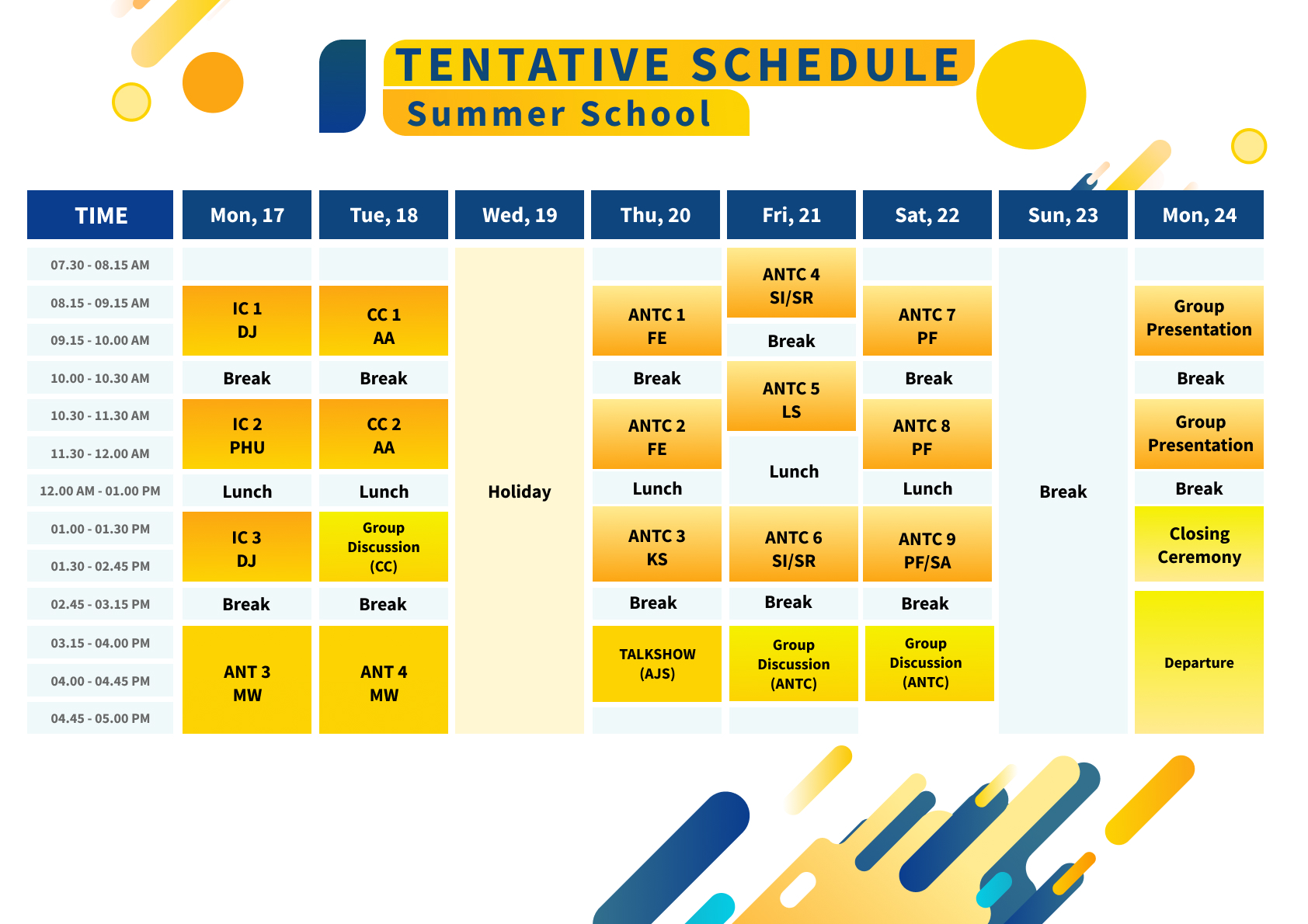### Venue

Opening Program and Talkshow : Auditorium 7th floor, FMNS, UGM

All Lecture Sessions : Meeting Room 1, 3rd floor, Department of Mathematics Building, FMNS, UGM

### Schedule## Speakers:

1. Prof. Ling San (LS)
2. Prof. Michel Waldschmidt (MW)
3. Prof. Francesco Pappalardi (FP)
4. Prof. Khurram Shabbir (KS)
5. Prof. Shakir Ali (SA)
6. Dr. Santi Indarjani (SI)
7. Dr. Sri Rosdiana (SR)
8. Dr. Diah Junia Eksi Palupi (DJ)
9. Dr. Toh Pee Choon (TPC)
10. Hong Nankun, Ph.D. (HN)
11. Zafer Selcuk Aygin, Ph.D. (ZSA)
12. Frederic Ezerman, Ph.D. (FE)
13. Wang Liuquan, Ph.D. (WL)
14. Dr. Richard Griffon (RG)
15. Putranto Hadi Utomo, Ph.D. (PHU)
16. Prastudy Fauzi, Ph.D. (PF)
18. Dr. Budi Surodjo (BS)
20. Uha Isnaini, Ph.D. (UI)

## Courses:

• Elementary Number Theory (ENT)

﻿Divisibility, Primality, Congruence, Extended Euclidean Algorithm, Chinese Remainder Theorem, Fermat Little Theorem, Charmicael number, Euler Phi Function, Quadratic Residue, Jacobi Symbol, Introduction to Elliptic Curve

• Introduction to Integer Partitions (IIP)

Partitions, Special type of Partitions, Frobenius Partitions, Rank, Crank, Introduction to Research in Partitions,

• Analytic Number Theory (ANT)

Riemann zeta function, Introduction to the Euler Gamma function, Classical theory of the distribution of primes, Analytic continuation and the functional equation of the Riemann zeta function, Review of Riemann’s seminal paper of 1859, Riemann Hypothesis

• Introduction to Cryptography (IC)

﻿Encryption, Decryption, Permutation Cipher, Hill Cipher, Hash Function, RSA, Diffie-Hellman, El Gamal, Code based cryptography, Block Cipher, Stream Cipher, Digital Signature

• Computation in Cryptography (CC)

Algorithms of some basic cryptography (RSA, Hill Cipher, Permutation cipher, Diffie-Hellman, El Gamal), Overview of brute force attack

• Application of Number Theory in Cryptography (ANTC)

Algebraic Attack, Linear and Differential Attack, Grobner Base, Security notions and primitives in pairing-based cryptography, Privacy-preserving techniques: zero-knowledge, homomorphic encryption, MPC, Introduction to post-quantum cryptography and its applications to preserving privacy